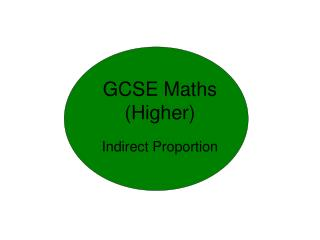DownloadDownload PresentationGCSE Maths (Higher)

# GCSE Maths (Higher)

Télécharger la présentation## GCSE Maths (Higher)

- - - - - - - - - - - - - - - - - - - - - - - - - - - E N D - - - - - - - - - - - - - - - - - - - - - - - - - - -
##### Presentation Transcript

1. GCSE Maths(Higher) Indirect Proportion

2. Types of proportion • Direct Proportion • percentage • Conversions • Ratio • Fractions • Similar shapes • Arc & sectors • How many more can you think of? More Less 70 60 mph Time (min) ? 90 Less More Less More Inverse Proportion Any question where increasing one number has the effect of reducing the other 8 50 km miles 5 ? Less More

3. What is number?Can you think of any more? Number Time Ratio Fractions Lengths Number Weights Money Percentages Standard Index Form

4. Below is a good rule of thumb for questions on proportion. Number Number Area (squarenumber) Area (square number) Volume (cube number) Volume (cube number)

5. Relationships that don’t fit No Number Number No Area (squarenumber) Area (square number) Volume (cube number) Volume (cube number) No

6. But what do you do when you are given a questions that don’t seem to fit? Number Number Area (squarenumber) Area (square number) Volume (cube number) Volume (cube number)

7. A gift shop sells small candles that are 8cm high and cost £3.20. It sells similar candles that are 14cm high. Number No Volume (cube number) What price are the large candles, if the cost of thecandleis proportional to its volume?

8. Strategy – extract information onto 3 lines 3.20 ? Cost 8 14 Height cm 512 2744 Height cm3 Large candles cost £17.15 in the gift shop A gift shop sells small candles that are 8cm high and cost £3.20. It sells similar candles that are 14cm high. What price are the large candles, if the cost of thecandleis proportional to its volume (not its height)?

9. No Number Area (square number) A gift shop sells small candles that are 8cm high and cost £3.20. It sells similar candles that are 14cm high. It takes 144cm2 of plastic film to wrap the small candle. How much does the large candle need?

10. Strategy extract information onto 3 lines 144 ? area 8 14 Height cm 64 196 Height cm2 Large candles need 441cm2 of wrap A gift shop sells small candles that are 8cm high and cost £3.20. It sells similar candles that are 14cm high. It takes 144cm2 of plastic film to wrap the small candle. How much does the large candle need?

11. Extract information from question onto lines. Question A simple pendulum of length L cm, takes T seconds to swing from A to B. T is proportional to square root of L. When T = 34 sec, L = 72.25cm • Express T in terms of L • Find T when L = 400 • Find L when T = 60 Calculation – to get method marks. a) Answer – make sure it is in context with the question a) T in terms of L is: T= 4 Strategy for Indirect proportion 72.25 L Lcm √72.25 √L √L 34 T T = sec

12. Extract information from question onto lines. Question A simple pendulum of length L cm, takes T seconds to swing from A to B. t is proportional to square root of L. When T = 34 sec, L = 72.25cm • Express T in terms of L • Find T when L = 400 • Find L when T = 60 Calculation – to get method marks. b) From a) Answer – make sure it is in context with the question a) T in terms of L is: T= 4 b) T=80 Strategy for Indirect proportion 72.25 L Lcm √72.25 √L √l 34 T T = sec

13. Extract information from question onto lines. Question A simple pendulum of length L cm, takes T seconds to swing from A to B. T is proportional to square root of L. When T = 34 sec, L = 72.25cm • Express T in terms of L • Find T when L = 400 • Find L when T = 60 Calculation – to get method marks. c) Answer – make sure it is in context with the question a) T in terms of L is: T= 4 b) T=80 when L = 400 c) L = 225 when T=60 Strategy for Indirect proportion 72.25 L Lcm √72.25 √L √l 34 T T = sec

14. Extract information from question onto lines. Question Calculation – to get method marks. Answer – make sure it is in context with the question Strategy for Indirect proportion